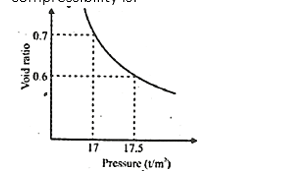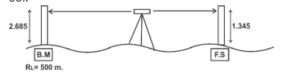Engineering Jobs   »   Civil Engineering quizs   »   DFCCIL QUIZ

# DFCCIL’21 CE: Daily Practice Quiz. 17-September-2021

DFCCIL (Dedicated Freight Corridor Corporation of India Limited) Exam Date 2021 on September and October 2021 so boost your preparation by attempt this Civil Engineering MISCELLANEOUS quiz for DFCCIL recruitment 2021.

Quiz: Civil Engineering
Exam: DFCCIL-JE
Topic: MISCELLANEOUS

Each question carries 1 mark
Negative marking: 1/4 mark
Time: 8 Minutes

Q1. The back staff reading on bench mark of R.L. 500.00 m is 2.685m. if foresight reading on the point is 1.345m, the reduced level of the point is
(a) 502.68 m
(b) 501.315 m
(c) 501.340 m
(d) 504.030 m

Q2. The sensitivity of a bubble tube can be increased by:
(a) increase the diameter of the tube
(b) Decreasing the length of the bubble
(c) Increasing the viscosity of liquid
(d) Decreasing the radius of curvature of the tube

Q3. A single acting steam hammer weighting 2000 kg and falling through a height of 2m drives a pile an average penetration of 0.5 cm under the last few blows. What will be the allowable load (in tonnes) for the pile?
(a) 40
(b) 80
(c) 88.88
(d) 92.44

Q4. Negative skin friction is a downward drawn acting on a pile due to
(b) the downward movement of the surrounding
(c) the upward movement of the surrounding
(d) none of these

Q5. The void ratio pressure diagram is shown in the given figure. The coefficient of compressibility is:(a) 0.0050 m²/t
(b) 0.073 m²/t
(c) 0.20 m²/t
(d) 0.25 m²/t

Q6. When the degree of consolidation is 50% about how much is the time factor?
(a) 0.5
(b) 2.0
(c) 1.0
(d) 0.2

SOLUTION

S1. Ans.(c)
Sol.Hight of instrument = 500+2.685
= 502.685 m.
RL of point = 502.685-1.345
= 501.34 m

S2. Ans.(a)
Sol. Sensitivity of the bubble tube/level tube can be increased by.
(ii) Increasing length of tube
(iii) Smoothing the inner surface of tube
(iv) Decreasing the viscosity of fluid inside tube
(v) decreasing surface tension of fluid inside tube

S3. Ans.(c)
Sol. W = 2000 kg
H = 2m.
S = 0.5 cm.
C = 0.25 cm (for steam hammer)
Q_up=WH/(S+C)
Q_up=(2000×200)/(0.5+0.25)
Q_up=533.33 ton
Q_allowable=Q_up/(f.O.S )
=533.33/6
=88.88 ton

S4. Ans.(b)
Sol. Negative skin friction is a downward drawn acting on a pile due to the downward movement of the surrounding.

S5. Ans.(c)
Sol. Coefficient of compressibility = |∆e/(∆σ ̅ )|
=|(0.7-0.6)/(17.5-17)|
=|0.1/0.5|=0.20 m^2 \/t

S6. Ans.(d)
Sol. When %U < 60%
time factor (T_v )=π/4 (%U)^2
T_V=3.14/4 (0.50)^2
▭(T_v=0.19625)
▭(T_v≈0.2)

Sharing is caring!

Thank You, Your details have been submitted we will get back to you.